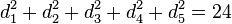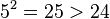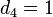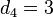# Determination of character table of symmetric group:S4

This page describes the process used for the determination of specific information related to a particular group. The information type is character table and the group is symmetric group:S4.
View all pages that describe how to determine character table of particular groups | View all specific information about symmetric group:S4

This page describes various ways to determine the character table of symmetric group:S4. The idea is to determine as much as possible wit has little knowledge of the representation theory of these groups as we can manage, therefore making the discussion suitable for people who know only basic facts about the groups and basic facts of linear representation theory. For a detailed discussion of the character theory, see linear representation theory of symmetric group:S4.

We build on the basic information on conjugacy class structure available at element structure of symmetric group:S4.

## Finding the degrees of irreducible representations using number-theoretic constraints

Final answer: The degrees of irreducible representations are 1, 1, 2, 3, 3.

The following facts are known in general:

1. Sum of squares of degrees of irreducible representations equals order of group: In this case, it tells us that the sum of the degrees of irreducible representations is 24, the order of the group.
2. Number of irreducible representations equals number of conjugacy classes: In this case, it tells us that there are 5 irreducible representations, because there are 5 conjugacy classes.
3. Degree of irreducible representation divides order of group: In this case, it tells us that the degrees of irreducible representations all divide 24, the order of the group.
4. Number of one-dimensional representations equals order of abelianization: In this case, the derived subgroup is A4 in S4 (alternating group:A4, order 12) and the abelianization (the quotient group) is isomorphic to cyclic group:Z2. So, the number 1 occurs two times as a degree of irreducible representation.
5. Order of inner automorphism group bounds square of degree of irreducible representation

In fact, we do not need all these facts to determine the degrees of irreducible representations. Some combinations of subsets of these facts suffice. For instance:

### Using (1) and (2) alone

(2) tells us that there are five irreducible representations. Denote their (positive integer) degrees, in non-decreasing order, as$d_1 \le d_2 \le \dots \le d_5$. (1) tells us that$d_1^2 + d_2^2 + d_3^2 + d_4^2 + d_5^2 = 24$. Note that$d_5 \le 4$ since$5^2 = 25 > 24$.

#### Case$d_5 = 4$

In this case, we obtain that$d_1^2 + d_2^2 + d_3^2 +d_4^2 = 24 - 4^2 = 8$. In other words, we need to express 8 as a sum of four squares of positive integers. This forces$d_4 \le 2$. Consider the cases$d_4 = 1$ and$d_4 = 2$:

•$d_4 = 2$. We thus obtain$d_1^2 + d_2^2 + d_3^2 = 4$. But there are no three positive integers whose sum of squares is 4. Therefore, this case does not work.
•$d_4 = 1$, then$d_1 = d_2 = d_3 = d_4 = 1$, leading to sum of squares 4 instead of 8. So this case does not work.

#### Case$d_5 = 3$

In this case, we obtain$d_1^2 + d_2^2 + d_3^2 + d_4^2 = 24 - 3^2 = 15$. We have$d_4 \le 3$. We make cases:

•$d_4 = 3$: In this case,$d_1^2 + d_2^2 + d_3^2 = 6$. The only solution to that is$d_1 = 1, d_2 = 1, d_3 = 2$. This gives the solution$d_1 = 1, d_2 = 1, d_3 = 2, d_4 = 3, d_5 = 3$.
•$d_4 = 2$: In this case,$d_1^2 + d_2^2 + d_3^2 = 11$, with$d_3 \le 2$ (because$d_3 \le d_4$). There are no solutions to this.
•$d_4 = 1$: In this case,$d_1 = d_2 = d_3 = 1$, which clearly does not work.

#### Case$d_5 \le 2$

In this case,$d_1,d_2,d_3,d_4,d_5$ are all at most 2, so the sum of squares is at most 20, falling short of the target of 24.

#### Upshot

The only solution is$d_1 = 1, d_2 = 1, d_3 = 2, d_4 = 3, d_5 = 3$.